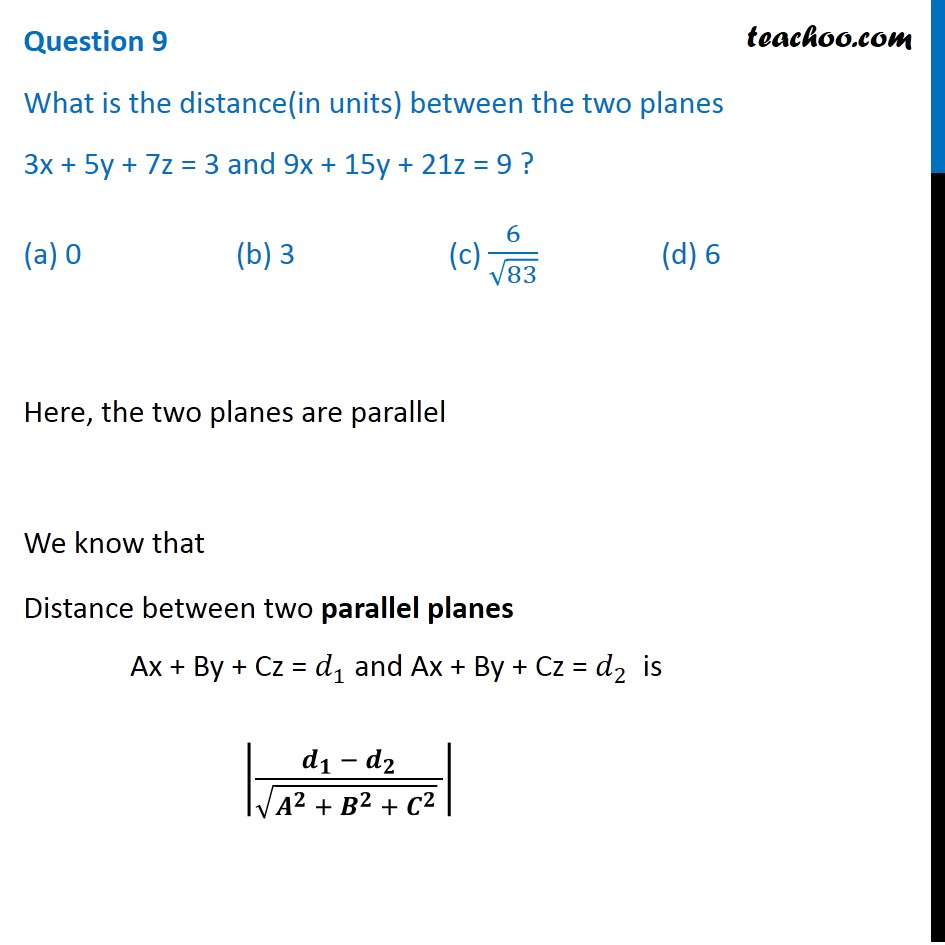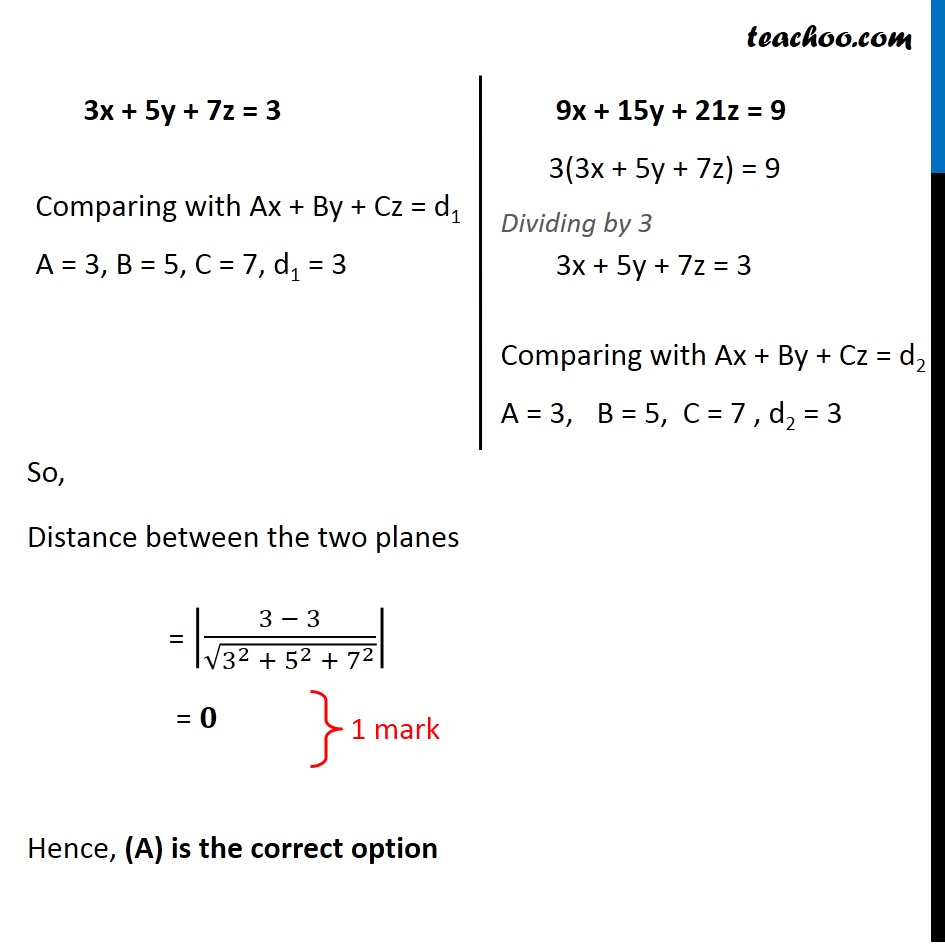CBSE Class 12 Sample Paper for 2020 Boards

Class 12
Solutions of Sample Papers and Past Year Papers - for Class 12 Boards

## (a) 0             (b) 3             (c) 6/√83               (d) 6Note : - This is similar to Misc 22 of NCERT – Chapter 11 Class 12

Learn in your speed, with individual attention - Teachoo Maths 1-on-1 Class

### Transcript

Question 9 What is the distance(in units) between the two planes 3x + 5y + 7z = 3 and 9x + 15y + 21z = 9 ? (a) 0 (b) 3 (c) 6/√83 (d) 6 Here, the two planes are parallel We know that Distance between two parallel planes Ax + By + Cz = 𝑑_1 and Ax + By + Cz = 𝑑_2 is |(𝒅_𝟏 − 𝒅_𝟐)/(√(𝑨^𝟐 + 𝑩^𝟐 + 𝑪^𝟐 ) )| 3x + 5y + 7z = 3 Comparing with Ax + By + Cz = d1 A = 3, B = 5, C = 7, d1 = 3 9x + 15y + 21z = 9 3(3x + 5y + 7z) = 9 Dividing by 3 3x + 5y + 7z = 3 Comparing with Ax + By + Cz = d2 A = 3, B = 5, C = 7 , d2 = 3 So, Distance between the two planes = |(3 − 3)/√(3^2 + 5^2 + 7^2 )| = 𝟎 }1 mark Hence, (A) is the correct option